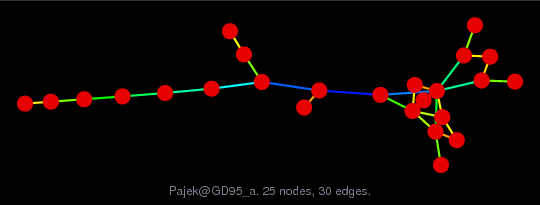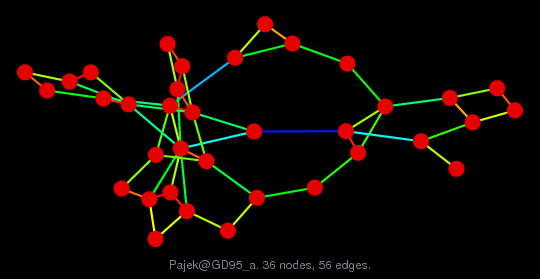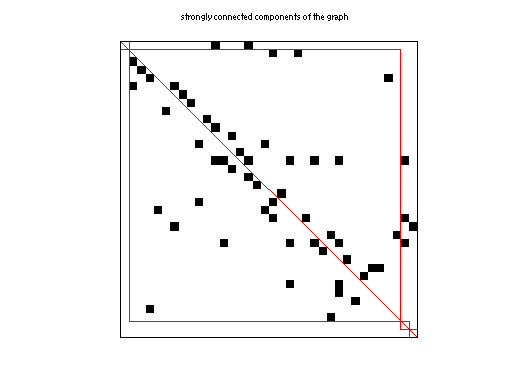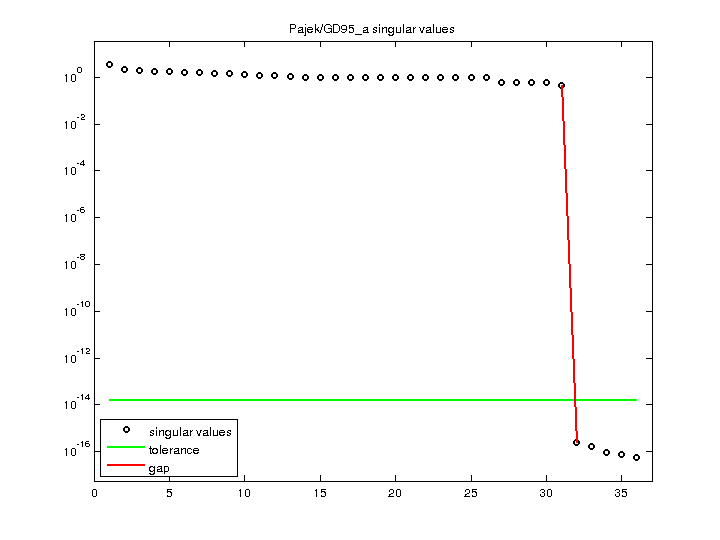Matrix: Pajek/GD95_a

Description: Pajek network: Graph Drawing contest 1995(bipartite graph drawing) (graph drawing of A+A')• Matrix group: Pajek
• download as a MATLAB mat-file, file size: 1 KB. Use UFget(1485) or UFget('Pajek/GD95_a') in MATLAB.

 Matrix properties number of rows 36 number of columns 36 nonzeros 57 # strongly connected comp. 4 explicit zero entries 0 nonzero pattern symmetry 4% numeric value symmetry 4% type binary structure unsymmetric Cholesky candidate? no positive definite? no

 author Graph Drawing Contest editor V. Batagelj date 1995 kind directed graph 2D/3D problem? no

 Additional fields size and type nodename full 36-by-31 coord full 36-by-2

Notes:

```------------------------------------------------------------------------------
Pajek network converted to sparse adjacency matrix for inclusion in UF sparse
matrix collection, Tim Davis.  For Pajek datasets, See V. Batagelj & A. Mrvar,
------------------------------------------------------------------------------
The original problem had 3D xyz coordinates, but all values of z were equal
to 0, and have been removed.  This graph has 2D coordinates.
```

 SVD-based statistics: norm(A) 3.55903 min(svd(A)) 5.5709e-17 cond(A) 6.3886e+16 rank(A) 31 null space dimension 5 full numerical rank? no singular value gap 1.84048e+15

 singular values (MAT file): click here SVD method used: s = svd (full (A)) status: ok